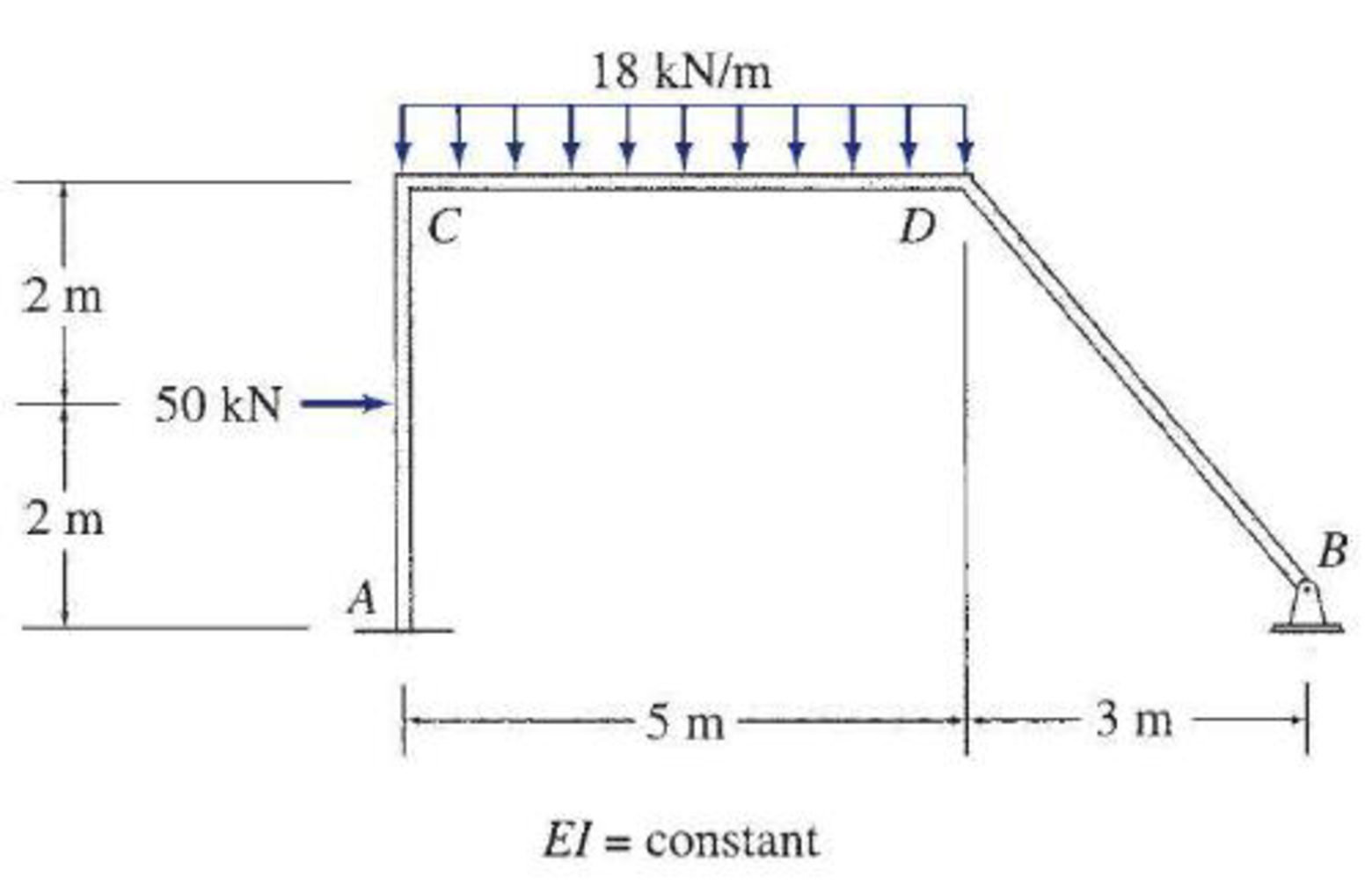# Determine the member end moments and reactions for the frames shown in Figs. P16.24–P16.31 by using the moment-distribution method. FIG. 16.29

#### Solutions

Chapter
Section
Chapter 16, Problem 29P
Textbook Problem
221 views

## Determine the member end moments and reactions for the frames shown in Figs. P16.24–P16.31 by using the moment-distribution method.FIG. 16.29

To determine

Find the member end moments and reactions for the frames.

### Explanation of Solution

Fixed end moment:

Formula to calculate the relative stiffness for fixed support IL and for roller support (34)(IL).

Formula to calculate the fixed moment for point load with equal length are PL8.

Formula to calculate the fixed moment for point load with unequal length are Pab2L2 and Pa2bL2.

Formula to calculate the fixed moment for UDL is WL212.

Formula to calculate the fixed moment for UVL are WL230 and WL220.

Formula to calculate the fixed moment for deflection is 6EIΔL2

Calculation:

Consider the flexural rigidity EI of the frame is constant.

Show the free body diagram of the entire frame as in Figure 1.

Refer Figure 1,

Calculate the length of the member AC:

LDB=32+42=9+16=5m

Calculate the relative stiffness KCA for member AC of the frame as below:

KCA=I4

Calculate the relative stiffness KCD for member CD of the frame as below:

KCD=I5

Calculate the relative stiffness KDC for member CD of the frame as below:

KDC=I5

Calculate the relative stiffness KDB for member BD of the frame as below:

KDB=(34)I5=3I20

Calculate the distribution factor DFCA for member CA of the frame.

DFCA=KCAKCA+KCD

Substitute I4 for KCA and I5 for KCD.

DFCA=I4I4+I5=0.556

Calculate the distribution factor DFCD for member CD of the frame.

DFCD=KCDKCA+KCD

Substitute I4 for KCA and I5 for KCD.

DFCD=I5I4+I5=0.444

Check for sum of distribution factor as below:

DFCA+DFCD=1

Substitute 0.556 for DFCA and 0.444 for DFCD.

0.556+0.444=1

Hence, OK.

Calculate the distribution factor DFDC for member DC of the frame.

DFDC=KDCKDC+KDB

Substitute I5 for KDC and 3I20 for KDB.

DFDC=I5I5+3I20=0.571

Calculate the distribution factor DFDB for member DB of the frame.

DFDB=KDBKDC+KDB

Substitute I5 for KDC and 3I20 for KDB.

DFDB=3I20I5+3I20=0.429

Check for sum of distribution factor as below:

DFDC+DFDB=1

Substitute 0.571 for DFDC and 0.429 for DFDB.

0.571+0.429=1

Hence, OK.

Calculate the fixed end moment for AC.

FEMAC=50×(4)8=25kNm

Calculate the fixed end moment for CA.

FEMCA=50×(4)8=25kNm

Calculate the fixed end moment for CD.

FEMCD=18×(5)212=37.5kNm

Calculate the fixed end moment for DC.

FEMDC=18×(5)212=37.5kNm

Calculate the fixed end moment for DB and BD.

FEMDB=FEMBD=0

Show the calculation of M0 moments using moment distribution method for side-sway prevented as in Table 1.

Show the free body diagram of the member AC, CD and DB for side-sway prevented as in Figure 2.

Consider member CD:

Calculate the vertical reaction at the joint C by taking moment about point D.

+MD=0Cy1(5)+(18×5×52)+38.718.5=0Cy1(5)=245.2Cy1=245.25Cy1=49.04kN

Calculate the vertical reaction at joint D by resolving the horizontal equilibrium.

+Fy=0Cy1+Dy1(18×5)=049.04+Dy190=0Dy1=40.96kN

Consider member AC

Calculate vertical reaction at joint A using the relation:

Ay1=Cy1=49.04kN

Calculate horizontal reaction at joint A by taking moment about point C.

+MC=0Ax1(4)+(50×2)+18.138.7=0Ax1(4)=79.4Ax1=79.44Ax1=19.85kN

Calculate the horizontal reaction at joint C by resolving the horizontal equilibrium.

+Fx=0Ax1+Cx1+50=019.85+Cx1+50=0Cx1=30.15kN

Consider member DB:

Calculate vertical reaction at joint B:

By1=Dy1=40.96kN

Calculate horizontal reaction at joint B by taking moment about point D.

+MD=0Bx1(4)+(40.96×3)+18.5+0=0Bx1(4)=141.38Bx1=141.384Bx1=35.35kN

Calculate the horizontal reaction at joint D by resolving the horizontal equilibrium.

+Fx=0Bx1+Dx1=035.35+Dx1=0Dx1=35.35kN

Show the unknown load R as in Figure 3.

Calculate the reaction R:

R=Cx1+Dx1=30.15+(35.35)=5.2kN

Show the arbitrary translation as in Figure 4.

Calculate the relative translation ΔAC between the ends of member AC:

ΔAC=Δ

Calculate the relative translation ΔCD between the ends of member CD:

ΔCD=(34)Δ=0.75Δ

Calculate the relative translation ΔBD between the ends of member BD:

ΔBD=(54)Δ=1.25Δ

Calculate the fixed end moment for AC and CA.

FEMAC=FEMCA=6EI(ΔAC)(4)2

Substitute Δ for ΔAC.

FEMAC=FEMCA=6EI(Δ)(4)2 (1).

Calculate the fixed end moment for CD and DC.

FEMCD=FEMDC=6EI(ΔCD)(5)2

Substitute 0.75Δ for ΔCD.

FEMCD=FEMDC=6EI(0.75Δ)(5)2        (2)

Calculate the fixed end moment for BD and DB.

FEMBD=FEMDB=6EI(ΔBD)(5)2

Substitute 1.25Δ for ΔBD.

FEMBD=FEMDB=6EI(1.25Δ)(5)2        (3)

Assume the Fixed-end moment at AC, and CA as 100kNm

FEMAC=FEMCA=100kNm

Calculate the value of EIΔ using the equation (1).

Substitute 100kNm for FEMAB

### Still sussing out bartleby?

Check out a sample textbook solution.

See a sample solution

#### The Solution to Your Study Problems

Bartleby provides explanations to thousands of textbook problems written by our experts, many with advanced degrees!

Get Started

Find more solutions based on key concepts
Same as Problem 8.6-2, but use ASD.

Steel Design (Activate Learning with these NEW titles from Engineering!)

Assuming an average 35% efficiency for power plants and a heating value of approximately 7.5 MJ/kg, calculate t...

Engineering Fundamentals: An Introduction to Engineering (MindTap Course List)

What is a subtype discriminator? Give an example of its use.

Database Systems: Design, Implementation, & Management

Desktop Operating Systems You are an analyst for a large manufacturer of laundry soaps. The company currently u...

Enhanced Discovering Computers 2017 (Shelly Cashman Series) (MindTap Course List)

What is a solid state storage device?

Principles of Information Systems (MindTap Course List)

Which terminal emulation protocol is similar to RDP but is open source?

Network+ Guide to Networks (MindTap Course List)

What is projectitis? How is it cured or its impact minimized?

Principles of Information Security (MindTap Course List)

What are three typical reasons why companies develop their own information systems?

Systems Analysis and Design (Shelly Cashman Series) (MindTap Course List)

Solve Problem 7.7-9 by using Mohr’s circle for plane strain.

Mechanics of Materials (MindTap Course List)

If your motherboard supports ECC DDR3 memory, can you substitute non-ECC DDR3 memory?

A+ Guide to Hardware (Standalone Book) (MindTap Course List)

After a weld is back gouged and a groove is formed, what should be done?

Welding: Principles and Applications (MindTap Course List)